Courses

# Long Answer Type Questions- Some Applications of Trigonometry Class 10 Notes | EduRev

## Class 10 : Long Answer Type Questions- Some Applications of Trigonometry Class 10 Notes | EduRev

The document Long Answer Type Questions- Some Applications of Trigonometry Class 10 Notes | EduRev is a part of the Class 10 Course Mathematics (Maths) Class 10.
All you need of Class 10 at this link: Class 10

Q1. A boy whose eye level is 1.3 m from the ground, spots a balloon moving with the wind in a horizontal level at some height from the ground. The angle of elevation of the balloon from the eyes of the boy at any instant is 60°. After 2 seconds, the angle of elevation reduces to 30°. If the speed of the wind at that moment is 29√3 m/s, then find the height of the balloon from ground.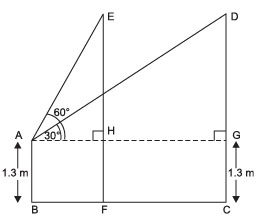Sol. Let E and D be the two positions of the balloon.

Let AB be the position of the boy.
∴ AB = 1.3 m
⇒ HF = CG = 1.3 m
Also speed of the wind = 29√3 m/s
Distance covered by the balloon in 2 seconds
= ED = HG = 2 × 29√3 m
= 58√3 m
∴ AG = AH + HG
= AH + 58√3 m ...(1)
Now, in right Δ AEH, we have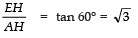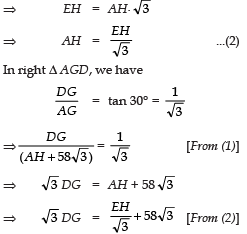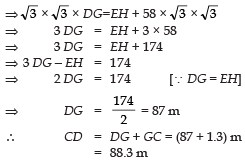Thus, the height of the balloon = 88.3 m.

Q2. From a point on the ground, the angles of elevation of the bottom and the top of a tower fixed at the top of a 20 m high building are 45° and 60° respectively. Find the height of the tower.

Sol. Let AB = 20 m be the building, P be the point on ground, and AC = xm be the tower.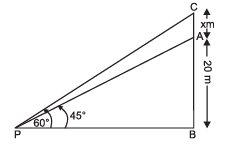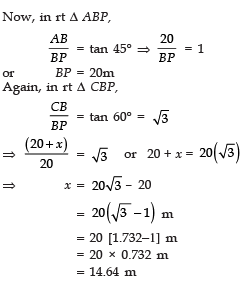Thus, the required height of the tower is 14.64 m

Q3. A statue, 1.5 m tall stands on the top of a pedestal. From a point on the ground, the angle of elevation of the top of the statue is 45° and from the same point the angle of elevation of the top of the pedestal is 30°.
Find the height of the pedestal from the ground.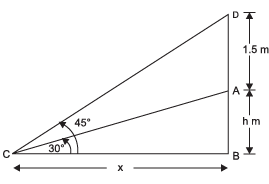Sol. Let AB be the pedestal and AB = h
Let C be the point on the ground such that
BC = x metres.
In right Δ ACB, we have: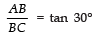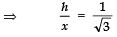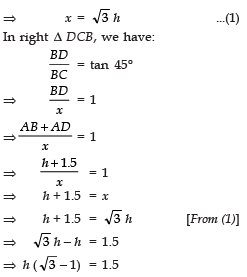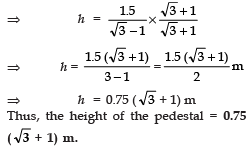Q4. The angles of depression of the top and battom of an 8 m tall building from the top of a multi-storeyed building are 30° and 45°, respectively. Find the height of the multistoreyed building and the distance between the two buildings.

Sol. Let the multistoreyed building be AB.
∴ AB = q metres
⇒ AD =(q − 8) m [∵ BD = 8 m]
Let EC be the small building.
Now, in right Δ ABC, we have: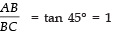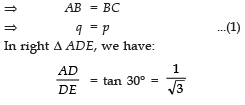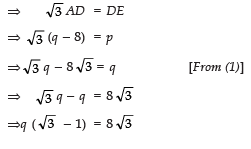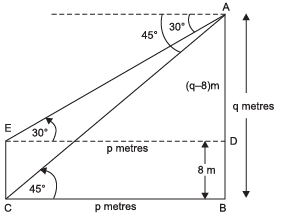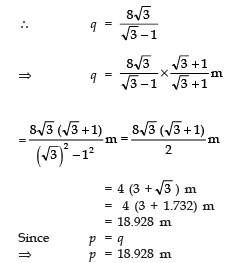∴ Distance between the two buildings = 18.928 m
Height of the multi-storeyed building = 18.928 m.

Q5. From the top of a building 60 m high, the angles of depression of the top and bottom of a vertical lamp post are observed to be 30° and 60° respectively. Find:

(i) The horizontal distance between the building and the lamp post.
(ii) The height of the lamp post.

[Take √3 = 1.732]

Sol. In the figure, let CE be the building and AB be the lamp post
∴ CE = 60 m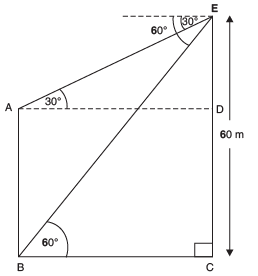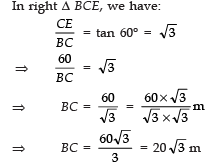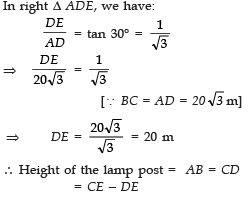= 60 m − 20
m = 40 m.
Also, the distances between the lamp post and the building
= 20√3 m = 20 × 1.732 m    [∵ 3 = 1.732]

= 34.64 m

Q6. The angle of elevation of a cloud from a point h meters above the surface of a lake is q and the angle of depression of its reflection in the lake is φ. Prove that the height of the clouds above the lake is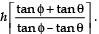Sol. Let P be the cloud and Q be its reflection in the lake. As shown in the figure, let A be the point of observation such that AB = h
Let the height of the cloud above the lake = x
Let AL = d
From rt ΔPLA,  tan θ =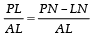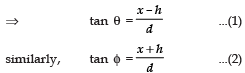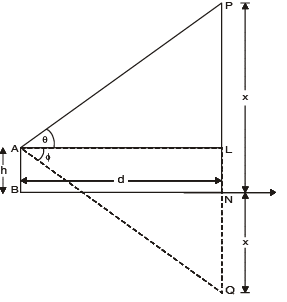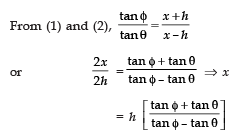Q7. From a point 100 m above a lake, the angle of elevation of a stationary helicopter is 30° and the angle of depression of reflection of the helicopter in the lake is 60°. Find the height of the helicopter.

Sol. In the figure, A is the stationary helicopter and F is its reflection in the lake.

In right Δ AED, we have: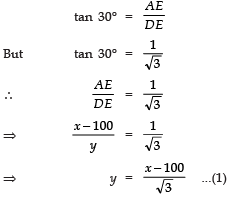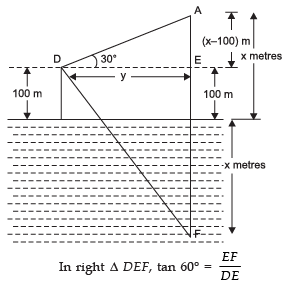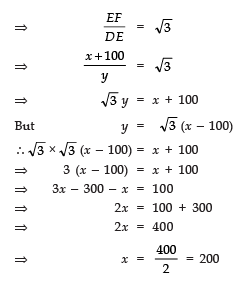Thus, the height of the stationary helicopter = 200 m.

Q8. The angle of elevation of an aeroplane from a point on the ground is 60°. After a flight of 15 seconds, the angle of elevation changes to 30°. If the aeroplane is flying at a constant height of 1500√3 m, find the speed of the aeroplane.

Sol. In the figure, let E and C be the two locations of the aeroplane.
Height BC = ED
= 1500√3 m

In right Δ ABC, we have: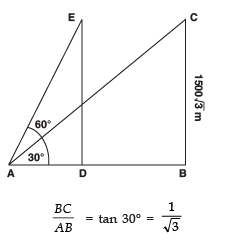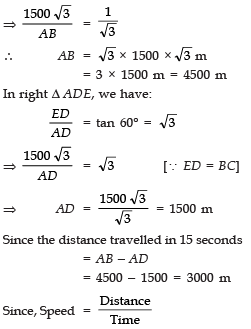∴ Speed of the aeroplane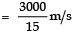= 200 m/s.

Q9. A spherical balloon of radius r subtends an angle q at the eye of the observer. If the angle of elevation of its centre is φ, find the heights of centre of the balloon.

Sol. In the figure, let O be the centre of the balloon, and A be the eye of the observer. r be the radius.

∴ OP = r and PAQ = θ
Also, ∠OAB = φ
Let the height of the centre of the balloon be ‘h’ ⇒ OB = h.
InΔOAP, ∠OPA = 90°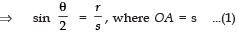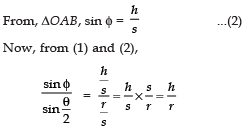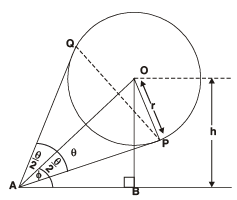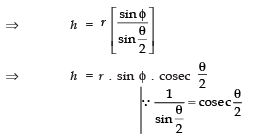Q10. As observered from the top of a light house, 100 m high above sea level, the angle of depression of a ship sailing directly towards it, changes from 30° to 60°. Determine the distances travelled by the ship during the period of observation. [Use√3 = 1.732]

Sol. Let A represents the position of the observer such that AB = 100 m
∴ In right Δ ABC, we have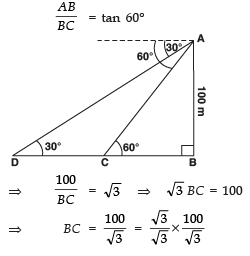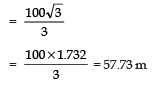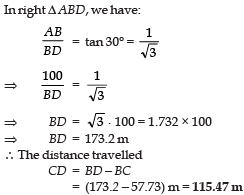Q11. From the top of a tower, 100 m high, a man observes two cars on the opposite sides of the tower with angles of depression 30° and 45° respectively. Find the distances between the cars. [use√3 = 1.73]

Sol. Let 'P' be the position of the observer.
A and B represent the cars. Height of the tower PQ = 100 m In rt Δ PQA,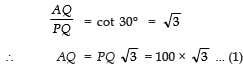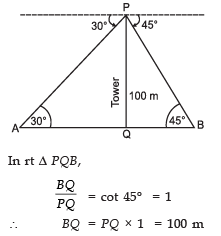Thus, distance between the cars A and B :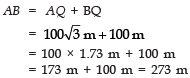Q12. The angles of elevation and depression of the top and the bottom of a tower from the top of a building, 60m high, are 30° and 60° respectively. Find the difference between the heights of the building and the tower and the distance between them.

Sol. Let AB is building = 60 m and DC is the tower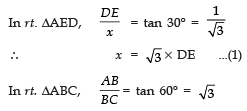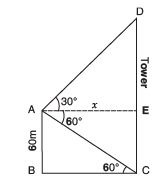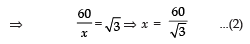Substituting the value of x from (2) in (1), we have :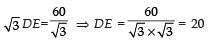⇒ Difference between the heights of building and tower = 20 m
Distance between the tower and building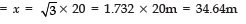Offer running on EduRev: Apply code STAYHOME200 to get INR 200 off on our premium plan EduRev Infinity!

## Mathematics (Maths) Class 10

178 videos|268 docs|103 tests

,

,

,

,

,

,

,

,

,

,

,

,

,

,

,

,

,

,

,

,

,

;